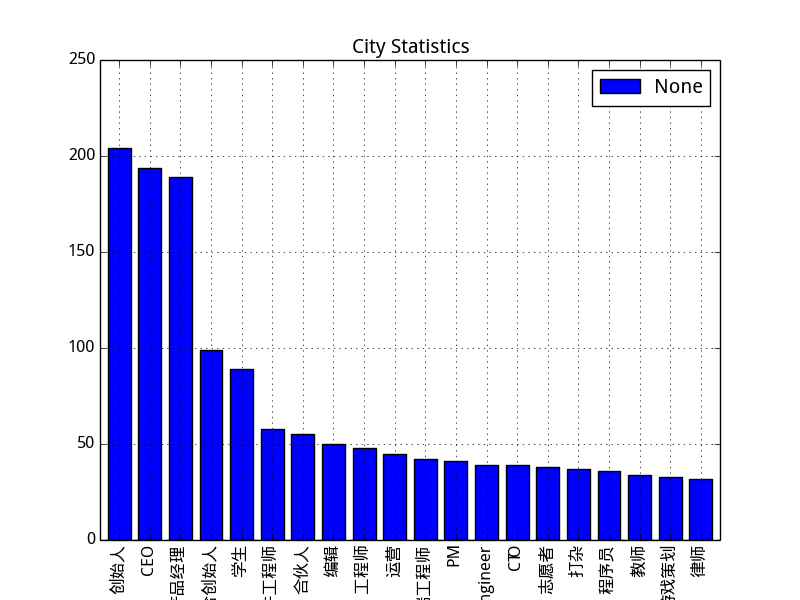python的pandas模块画图时中文显示不完全``````     target=pandas.read_csv('zhihu_user_data_30k.csv',encoding='utf-8')
city_count=target[u'职位'].value_counts()[:20]
plt=city_count.plot(kind='bar',title="City Statistics").get_figure()
mplt.legend(loc='best')
plt.savefig("city.png")

``````

1个回答

df.plot(kind=chart_type, title=title, fontsize=10, legend=True, rot=10)Python画图中文显示

【python】解决画图中文显示问题
pyplot画图时会产生乱码问题，类似这种： 在代码中加入： from pylab import * mpl.rcParams['font.sans-serif']=['SimHei'] 就可以显示中文了：
python 的pandas模块学习

python和pandas之时间序列模块
1.python中的时间序列模块，这里就不详细的说明，可以戳这个网址 python的datetime模块 2.pandas中时间序列模块 1）pandas通常用于处理成组日期，不管这些日期是DataFrame的轴索引还是列，to_datetime方法可以解析多种不同的日期表示形式。 &amp;gt;&amp;gt;&amp;gt;date = ['2017-6-26', '2017-6-27'] &amp;gt;&amp;gt...
pandas画图
Serise上的画图 import matplotlib.pyplot as plt import pandas as pd from pandas import * import numpy as np s = Series(np.random.rand(10).cumsum(),index=np.arange(0,100,10)) s.plot() plt
Python中的pandas模块学习
Python中的numpy模块学习 本文是基于Windows系统环境，学习和测试pandas模块： Windows 10 PyCharm 2018.3.5 for Windows (exe) python 3.6.8 Windows x86 executable installer 1. 读取csv/txt文件 读取txt文件，设置分隔符为‘，’，设置是否跳过第一行 impo...
【Python数据挖掘】实用模块之Pandas
Pandas模块是一个强大的数据分析和处理模块，能为复杂情形下的数据提供坚实的基础分析功能。 所谓的复杂情形，可能有以下三种： ①数据库表或Excel表，包含了多列不同数据类型的数据（如数字、文字） ②时间序列类型的数据，包括有序和无序的情形，甚至是频率不固定的情形 ③任意的矩阵型、二维表、观测统计数据，允许独立的行或列带有标签 下面介绍Pandas模块中的基本的高级数据结构，以
Python中pandas模块的使用方法

Pandas画图 4.4.1 pandas.DataFrame.plot DataFrame.plot(x=None, y=None, kind=‘line’) x : label or position, default None y : label, position or list of label, positions, default None Allows plotting of...
ubuntu python 画图使用中文显示文字
ubuntu python 画图使用中文显示文字查看系统中已有的中文字体 查看系统中已有的中文字体 fc -list #########会出现类似以下格式的内容######## /usr/share/fonts/truetype/arphic/ukai.ttc: AR PL UKai CN:style=Book /usr/share/fonts/truetype/arphic/ukai.ttc: ...

python中的matplotlib模块画图
matplotlib画图的主要过程如下程序所示： import matplotlib.pyplot as plt import numpy as np x = np.arange(0,1.1,0.1) y = x**2 y2 = x**2+0.1 print(x,y) # 修改参数 plt.rcParams['font.sans-serif'] = 'SimHei' # 仿宋字体 plt.rc...
Python数据处理之（十 七）Pandas plot画图

Python中turtle模块画图

python pandas读取Excel数据后用plotly画图
import pandas as pd import plotly.offline as py import plotly.graph_objs as go def chart(): excelFile = r'test.xlsx' df_excel = pd.DataFrame(pd.read_excel(excelFile, orient='index')).sort_in...
python 用pandas读取excel并画图

pandas模块
pandas模块的使用

import numpy as np import pandas as pd import matplotlib.pyplot as plt ser=pd.Series(np.random.randn(10).cumsum(),index=np.arange(0,100,10)) ser.plot(kind='line',figsize=(4,3),title='pandas_plot',grid...
（89）--Python数据分析：Pandas画图
# Pandas画图import itertools from math import exp import pandas as pd import numpy as np import matplotlib.pyplot as plt def lasso_regression(X_array, y, lambd, threshold=0.1): #通过坐标下降(coordinate de...
pandas画图的几个技巧
1、pandas画图的时候颜色种类不够 我们经常会用pandas处理数据，处理完之后，很多时候会画个图看看。但是pandas默认的曲线就只有十种颜色，如果我们要绘制的数据过多，就会出现颜色重复。其实很简单，pandas绘图的时候有一个colors参数，我们可以对这个参数进行赋值。 data_df.plot(figsize=(19, 10), colors=cnames.keys()) 其实...
[Python] 常用模块（5）：pandas 4
Python 常用模块系列： [Python] 常用模块（1）：内建模块 – random &amp; datetime [Python] 常用模块（2）：内建模块 – re 以及正则表达式 [Python] 常用模块（3）：内建模块 – math &amp; itertools [Python] 常用模块（4）：NumPy 上 [Python] 常用模块（4）：NumPy 中 [Python]...
Python的pandas模块索引与数据选择
Python的pandas模块索引与数据选择 对于入手Python机器学习和数据分析的新手来说，pandas是必须要学习的相关模块之一。其中最让人觉得比较乱的一个是索引的选取，另一个是axis轴向的问题。这篇文章先讲述数据选取的解决思路，以后再写axis轴向的问题。 笼统的说，pandas的数据选取体现在4个方面：选择列；选择行；选择一片区域；选择一个单元格的具体数据。 import numpy ...
[Python] 常用模块（5）：pandas 5
Python 常用模块系列： [Python] 常用模块（1）：内建模块 – random &amp; datetime [Python] 常用模块（2）：内建模块 – re 以及正则表达式 [Python] 常用模块（3）：内建模块 – math &amp; itertools [Python] 常用模块（4）：NumPy 上 [Python] 常用模块（4）：NumPy 中 [Python]...
[Python] 常用模块（5）：pandas 3
Python 常用模块系列： [Python] 常用模块（1）：内建模块 – random &amp; datetime [Python] 常用模块（2）：内建模块 – re 以及正则表达式 [Python] 常用模块（3）：内建模块 – math &amp; itertools [Python] 常用模块（4）：NumPy 上 [Python] 常用模块（4）：NumPy 中 [Python]...
[Python] 常用模块（5）：pandas 1
Python 常用模块系列： [Python] 常用模块（1）：内建模块 – random &amp; datetime [Python] 常用模块（2）：内建模块 – re 以及正则表达式 [Python] 常用模块（3）：内建模块 – math &amp; itertools [Python] 常用模块（4）：NumPy 上 [Python] 常用模块（4）：NumPy 中 [Python]...
Python数据处理——Pandas模块使用（五）

Python数据处理——Pandas模块使用（二）

Python时间序列处理之datetime与pandas模块

Python数据处理——Pandas模块使用（七）

python模块pandas的基本使用实例
pandas用处 pandas为处理数据分析任务而创建  适合处理序列数据、表格数据等具良好结构的数据 其中数据结构：from pandas import Series、DataFrame、Panel Series：一维数组，类似list，不过数组中元素数据类型相同，且索引不限于0~n（默认） DataFrame：二维表格型数据结构，即键值对型。Series的容器。可以像操作SQL一样。 ...
python学习(九)----pandas模块相关函数
pandas的基本数据结构
Pandas模块之Python批量处理Excel导入MySQL
最近由于比赛需求，需要大量的数据支持系统的功能测试。然后比较坑的是，所有的源数据都是来自Excel文件格式。虽然我们可以用Navicat Premium 12类似的工具导入，但是这类的工具似乎只有一个一个导入excel文件的功能，并不能满足我们有3600多张Excel表的需求呀。        于是就想到了我们伟大的python，可以说python这种语言用来处理批量数据实在是再好...
[Python] 常用模块（5）：pandas 2
Python 常用模块系列： [Python] 常用模块（1）：内建模块 – random &amp; datetime [Python] 常用模块（2）：内建模块 – re 以及正则表达式 [Python] 常用模块（3）：内建模块 – math &amp; itertools [Python] 常用模块（4）：NumPy 上 [Python] 常用模块（4）：NumPy 中 [Python]...

1.读csv文件数据 import pandas as pd Info = pd.read_csv('titanic_train.csv'); #print(type(Info)) #Info的类型 &amp;amp;lt;class 'pandas.core.frame.DataFrame'&amp;amp;gt; #Info.dtypes #得到每个字段的类型 字符串类型变为objec #help...
Python数据处理——Pandas模块使用（一）

[Python] 常用模块（5）：pandas 8
Python 常用模块系列： [Python] 常用模块（1）：内建模块 – random &amp; datetime [Python] 常用模块（2）：内建模块 – re 以及正则表达式 [Python] 常用模块（3）：内建模块 – math &amp; itertools [Python] 常用模块（4）：NumPy 上 [Python] 常用模块（4）：NumPy 中 [Python]...
[Python] 常用模块（5）：pandas 7
Python 常用模块系列： [Python] 常用模块（1）：内建模块 – random &amp; datetime [Python] 常用模块（2）：内建模块 – re 以及正则表达式 [Python] 常用模块（3）：内建模块 – math &amp; itertools [Python] 常用模块（4）：NumPy 上 [Python] 常用模块（4）：NumPy 中 [Python]...
Python学习------pandas模块整理笔记
Introduce:pandas is an open source, BSD-licensed library providing high-performance, easy-to-use data structures and data analysis tools for the Python programming language.(details transfer to http:...
[Python] 常用模块（5）：pandas 9
Python 常用模块系列： [Python] 常用模块（1）：内建模块 – random &amp; datetime [Python] 常用模块（2）：内建模块 – re 以及正则表达式 [Python] 常用模块（3）：内建模块 – math &amp; itertools [Python] 常用模块（4）：NumPy 上 [Python] 常用模块（4）：NumPy 中 [Python]...